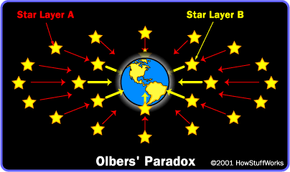# Why does the sky get dark at night?If Star Layer A is twice as far from Earth as Star Layer B, then the amount of light that reaches us from each star in A is only one-fourth the amount of light that reaches us from each star in B; but there are four times as many stars in A as there are in B.

The answer to this seemingly simple question may boggle your brain. It's actually a famous cosmological problem, formally known as Olbers' Paradox. (Heinrich Olbers was a German astronomer who popularized discussion of this subject in 1826.) You might think that the question can be explained away by the effect of distance -- not so. To fully understand the perplexity, picture stars of equal brightness distributed evenly in concentric layers around Earth, like shells around a nut. The same amount of light should reach Earth from each layer, because although the amount of light to reach us from each star decreases with distance (by 1/d^2), the number of stars in each layer increases, effectively balancing out the distance effect.If the distance between A and B is 2 units, then each square in A is one-fourth as bright as each square in B; but there are four times as many squares in A as there are in B.

So light lost to distance does not account for the darkness of night. Obscuration by dust is not the answer, either, as any dust in the path of light would heat up and eventually reradiate. Most modern cosmologists have settled on two theories to account for the darkness. The first one states that red shift (see Echo and Doppler Shift), which indicates that space itself is expanding, decreases the amount of light reaching us. The other explanation -- generally considered the main one -- is that the universe is not infinitely old. If it were, the sky would in fact be infinitely bright, because light from every point in the universe would have had time (eternity) to travel to every other point. As far as we know, there is no edge of the universe, only an edge of time. The finite age of the universe limits how much light we see.